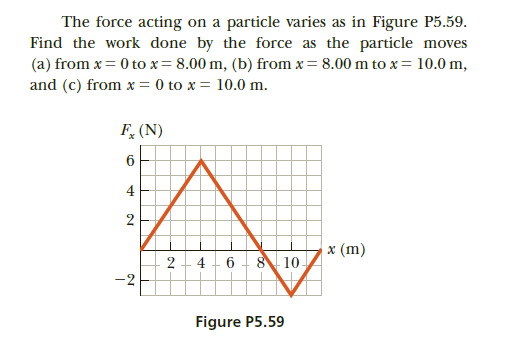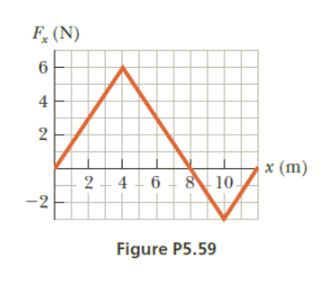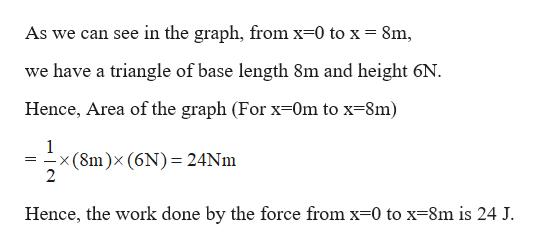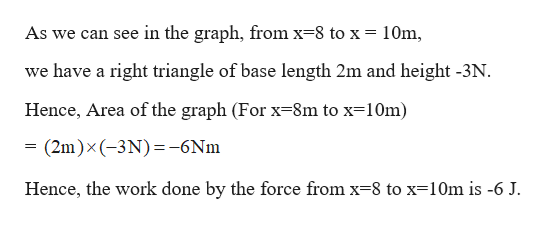# The force acting on a particle varies as in Figure P5.59.Find the work done by the force as the particle moves(a) from x = 0 to x= 8.00 m, (b) from x = 8.00 m to x= 10.0 m,and (c) from x = 0 to x = 10.0 m.F, (N)4x (m)10.2 - 4 - 6-2Figure P5.592.

Question
37 viewshelp_outlineImage TranscriptioncloseThe force acting on a particle varies as in Figure P5.59. Find the work done by the force as the particle moves (a) from x = 0 to x= 8.00 m, (b) from x = 8.00 m to x= 10.0 m, and (c) from x = 0 to x = 10.0 m. F, (N) 4 x (m) 10. 2 - 4 - 6 -2 Figure P5.59 2. fullscreen
check_circle

Step 1

Given:help_outlineImage TranscriptioncloseF, (N) 4 2 x (m) 2- 4 - 6 - 8 10- -2 Figure P5.59 fullscreen
Step 2

Calculating the work done by the force as the particle moves from x=0m to x=8m:

In a force-displacement graph, Work done is nothing but the area of the graph. The area above the x-axis is considered to be positive and the area below the x-axis is considered to be negative. Likewise, the Work done.help_outlineImage TranscriptioncloseAs we can see in the graph, from x=0 to x = 8m, we have a triangle of base length 8m and height 6N. Hence, Area of the graph (For x=0m to x=8m) <(8m)×(6N)= 24NM Hence, the work done by the force from x=0 to x=8m is 24 J. fullscreen
Step 3

Calculating the work dine by the force as the particle moves from x=8m to x=10m:

In a force-displacement graph, Work done is nothing but the area of the gr...help_outlineImage TranscriptioncloseAs we can see in the graph, from x=8 to x = 10m, we have a right triangle of base length 2m and height -3N. Hence, Area of the graph (For x=8m to x=10m) = (2m)x(-3N)=-6Nm Hence, the work done by the force from x=8 to x=10m is -6 J. fullscreen

### Want to see the full answer?

See Solution

#### Want to see this answer and more?

Solutions are written by subject experts who are available 24/7. Questions are typically answered within 1 hour.*

See Solution
*Response times may vary by subject and question.
Tagged in

### Physics